# Decrypting Data Using a Symmetric Provider

#### Microsoft Enterprise Library 5.0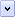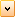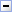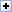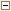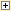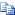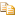If you encrypt data by using a symmetric encryption provider, you usually have to decrypt the data using the same provider.

#Typical Goals

In this scenario, you want use a symmetric provider to decrypt data that you provide. The output of the symmetric provider is the unencrypted data.

#Solution

Call the appropriate overload of the DecryptSymmetric method of the CryptographyManager class to perform the decryption, supplying the name of the configured symmetric provider to be used and the data that you want to decrypt as a string or a byte array.

#Using DecryptSymmetric

The following code shows how to use the DecryptSymmetric method to decrypt data in the form of a base-64 encoded string. These examples assume you have obtained an instance of the CryptographyManager class through the Enterprise Library container and saved it in the variable named crypto.

C#Copy Code
```string encryptedContentsBase64 = crypto.EncryptSymmetric("symmProvider", "password");

// Decrypt the base64 encoded string.
string readableString = crypto.DecryptSymmetric("symmProvider", encryptedContentsBase64);```
Visual BasicCopy Code
```Dim encryptedContentsBase64 As String = crypto.EncryptSymmetric("symmProvider", "password")

' Decrypt the base64 encoded string.
Dim readableString As String = crypto.DecryptSymmetric("symmProvider", encryptedContentsBase64)```

The following code shows how to use the DecryptSymmetric method to decrypt data in the form of a byte array.

C#Copy Code
```byte[] valueToEncrypt = Encoding.Unicode.GetBytes("password");
byte[] encryptedContents = crypto.EncryptSymmetric("symmProvider", valueToEncrypt);

byte[] decryptedContents = crypto.DecryptSymmetric("symmProvider", encryptedContents);
string plainText = (new UnicodeEncoding()).GetString(decryptedContents);```
Visual BasicCopy Code
```Dim valueToEncrypt = Encoding.Unicode.GetBytes("password")
Dim encryptedContents As Byte() = crypto.EncryptSymmetric("symmProvider", valueToEncrypt)

Dim decryptedContents As Byte() = crypto.DecryptSymmetric("symmProvider", encryptedContents)
Dim plainText As String = (New UnicodeEncoding()).GetString(decryptedContents)```

#Usage Notes

Make sure you configure the appropriate symmetric provider in the Enterprise Library configuration tools.